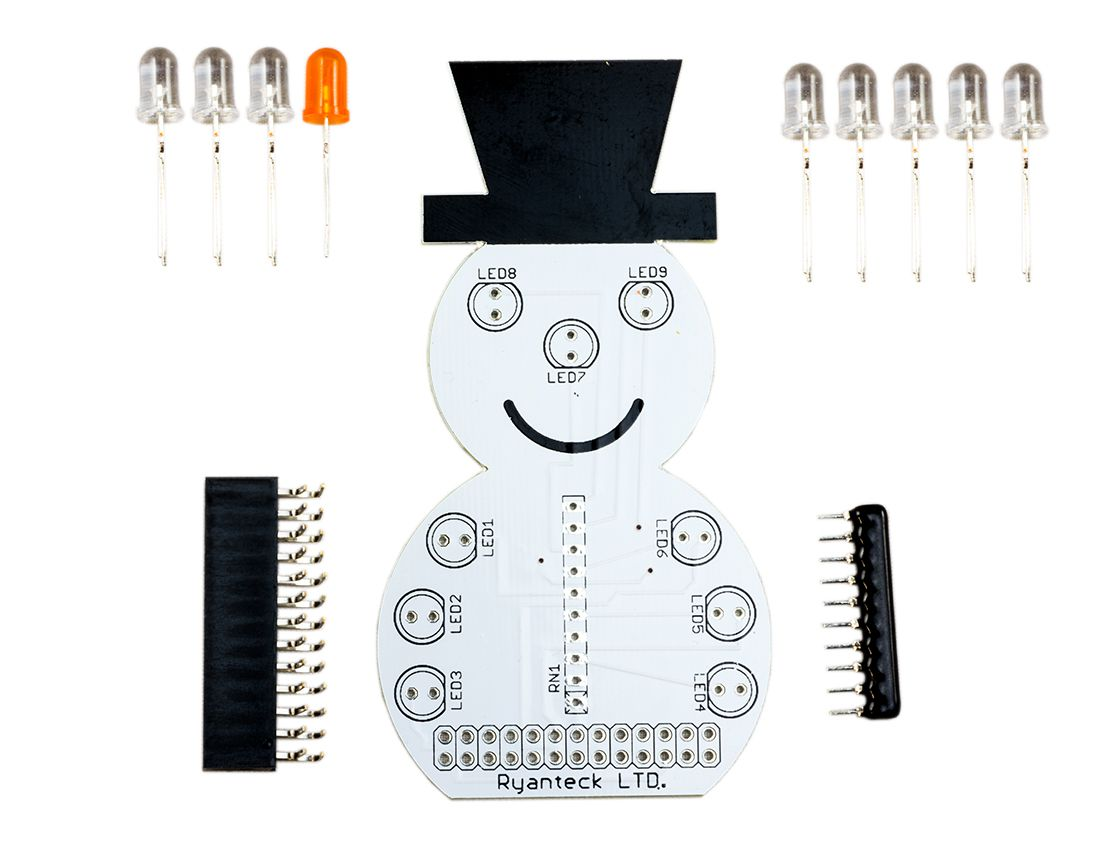# Ryanteck SnowPi - Demo 1

This is another demo program for the SnowPi written by Tony Goodhew.

A video tweet can be found at |@MrTomsWorld twitter.

```#!/usr/bin/python

# Tony Goodhew 19 November 2015

# Uses SnowPi and switch with 10K Ohm pull up on GPIO #16

# PWM brightness control of nose

# Import required libraries

import RPi.GPIO as GPIO

import time

import random

random.seed()

# Tell GPIO library to use GPIO references

GPIO.setmode(GPIO.BCM)

sw = 16

GPIO.setup(sw,GPIO.IN)

LEDs = [7,8,9,22,18,17,23,24] # Not nose

# Setup LED pins as outputs

for x in range(8):

GPIO.setup(LEDs[x], GPIO.OUT)

GPIO.output(LEDs[x], False)

# Light white and blue LEDs

for x in range(8):

GPIO.output(LEDs[x], True)

# Set up PWM on nose to control brightness

LED = 25

freq = 200 # PWM frequency - 200 times /sec

dutyCycle = 0 # percentage of time on - range 0 to 100

GPIO.setup(LED,GPIO.OUT)

pwmLED = GPIO.PWM(LED,freq)

pwmLED.start(0)

def spin():   #Clockwise

for i in range(6):

GPIO.output(LEDs[i],0) # White Off

for n in range(6):

for i in range(6):

GPIO.output(LEDs[i],1)

time.sleep(0.07)

GPIO.output(LEDs[i],0)

for i in range(6):

GPIO.output(LEDs[i],1) #White ON

return

def spin2():   # Counter Clockwise

for i in range(6):

GPIO.output(LEDs[i],0) # White OFF

for n in range(6):

for i in range(5,-1,-1):

GPIO.output(LEDs[i],1)

time.sleep(0.07)

GPIO.output(LEDs[i],0)

for i in range(6):

GPIO.output(LEDs[i],1) # White ON

return

def wink():

GPIO.output(LEDs,0)

time.sleep(0.2)

GPIO.output(LEDs,1)

return

def wink2():

GPIO.output(LEDs,0)

time.sleep(0.2)

GPIO.output(LEDs,1)

return

def upDown():                  # Up and Down

for i in range(6):

GPIO.output(LEDs[i],0) # White OFF

for i in range(4):

for n in range(3):

GPIO.output(LEDs[n],1)

GPIO.output(LEDs[5-n],1)

time.sleep(0.1)

GPIO.output(LEDs[n],0)

GPIO.output(LEDs[5-n],0)

time.sleep(0.1)

for m in range(3):

n = 2-m

GPIO.output(LEDs[n],1)

GPIO.output(LEDs[5-n],1)

time.sleep(0.1)

GPIO.output(LEDs[n],0)

GPIO.output(LEDs[5-n],0)

time.sleep(0.1)

for i in range(6):

GPIO.output(LEDs[i],1) # White ON

return

def wobble():                  # Side to side

for i in range(6):

GPIO.output(LEDs[i],0) # White OFF

for i in range(6):

GPIO.output(7,1)

GPIO.output(8,1)

GPIO.output(9,1)

time.sleep(0.1)

GPIO.output(7,0)

GPIO.output(8,0)

GPIO.output(9,0)

time.sleep(0.1)

GPIO.output(22,1)

GPIO.output(18,1)

GPIO.output(17,1)

time.sleep(0.1)

GPIO.output(22,0)

GPIO.output(18,0)

GPIO.output(17,0)

time.sleep(0.1)

for i in range(6):

GPIO.output(LEDs[i],1) # White ON

return

# ==== Main begins ====

pwmLED.ChangeDutyCycle(100) # Nose full brightness

wobble()

upDown()

spin()

wink()

spin2()

wink2()

print("Hold button down to stop")

# === Loop ===

swVal = GPIO.input(sw)

while swVal == 1:

for brite in range(0,90,5):

pwmLED.ChangeDutyCycle(brite) # Brighter nose

time.sleep(0.1)

n = random.randint(0,5)

if n == 0:

spin()

if n == 1:

spin2()

if n == 2:

wink()

if n == 3:

wink2()

if n == 4:

wobble()

if n == 5:

upDown()

for brite in range(90,0,-5):

pwmLED.ChangeDutyCycle(brite) # Dimmer nose

time.sleep(0.1)

swVal = GPIO.input(sw) # Check switch for stopping

# === Tidy up ===

print("Halted by button")

# Reset GPIO settings

GPIO.cleanup()```